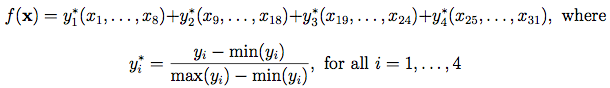Moon et al. (2012) FunctionDescription:

Dimensions: 31

This complex 31-dimensional function is created from a summation of scaled outputs from four other functions. For the purpose of input variable screening, 29 input variables are added, making it a 60-dimensional function.

Input Domain:

The input variables and their usual input ranges are:

Borehole Function:
 x1 ∈ [0.05, 0.15] radius of borehole (m) x2 ∈ [100, 50000] radius of influence (m) x3 ∈ [63070, 115600] transmissivity of upper aquifer (m2/yr) x4 ∈ [990, 1110] potentiometric head of upper aquifer (m) x5 ∈ [63.1, 116] transmissivity of lower aquifer (m2/yr) x6 ∈ [700, 820] potentiometric head of lower aquifer (m) x7 ∈ [1120, 1680] length of borehole (m) x8 ∈ [9855, 12045] hydraulic conductivity of borehole (m/yr)

Wing Weight Function:
 x9 ∈ [150, 200] wing area (ft2) x10 ∈ [220, 300] weight of fuel in the wing (lb) x11 ∈ [6, 10] aspect ratio x12 ∈ [-10, 10] quarter-chord sweep (degrees) x13 ∈ [16, 45] dynamic pressure a cruise (lb/ft2) x14 ∈ [0.5, 1] taper ratio x15 ∈ [0.08, 0.18] aerofoil thickness to chord ratio x16 ∈ [2.5, 6] ultimate load factor x17 ∈ [1700, 2500] flight design gross weight (lb) x18 ∈ [0.025, 0.08] paint weight (lb/ft2)

OTL Circuit Function:
 x19 ∈ [50, 150] resistance b1 (K-Ohms) x20 ∈ [25, 70] resistance b2 (K-Ohms) x21 ∈ [0.5, 3] resistance f (K-Ohms) x22 ∈ [1.2, 2.5] resistance c1 (K-Ohms) x23 ∈ [0.25, 1.2] resistance c2 (K-Ohms) x24 ∈ [50, 300] current gain (Amperes)

Piston Simulation Function:
 x25 ∈ [30, 60] piston weight (kg) x26 ∈ [0.005, 0.020] piston surface area (m2) x27 ∈ [0.002, 0.010] initial gas volume (m3) x28 ∈ [1000, 5000] spring coefficient (N/m) x29 ∈ [90000, 110000] atmospheric pressure (N/m2) x30 ∈ [290, 296] ambient temperature (K) x31 ∈ [340, 360] filling gas temperature (K)

References:

Moon, H., Dean, A. M., & Santner, T. J. (2012). Two-stage sensitivity-based group screening in computer experiments. Technometrics, 54(4), 376-387.

Additional reference information can be found at the links of the corresponding functions.